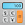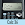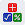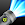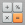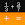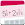# Fraction Calculator Plus

by Digitalchemy, LLC
10,000,000+ installs

Android application Fraction Calculator Plus developed by is listed under category Tools. According to Google Play Fraction Calculator Plus achieved more than 10,000,000 installs. Fraction Calculator Plus currently has 193,643 ratings with average rating value of 4.88. The current percentage of ratings achieved in last 30 days is 0.27%, percentage of ratings achieved in last 60 days is 0.4%. Fraction Calculator Plus has the current market position #5355 by number of ratings. A sample of the market history data for Fraction Calculator Plus can be found below. Last update on 2023-03-04.

Title: Fraction Calculator Plus Digitalchemy, LLC Tools Free Android
Total ratings: 193643 0.27% 0.4% 4.88
Installs (achieved): 10,000,000+ 11,911,068
5 star ratings: 181,983 6,262 1,656 911 2,820

2020-05-12: Android application Fraction Calculator Plus achieved 10,000,000 installs. Android application Fraction Calculator Plus achieved 5,000,000 installs. Android application Fraction Calculator Plus achieved 1,000,000 installs. Android application Fraction Calculator Plus achieved 500,000 installs. Android application Fraction Calculator Plus achieved 100,000 installs. Android application Fraction Calculator Plus achieved 50,000 installs. Android application Fraction Calculator Plus achieved 10,000 installs. Android application Fraction Calculator Plus achieved 5,000 installs.

Total number of ratings
Total number of active users rated for Fraction Calculator Plus.

Total number of installs (*estimated)
Estimation of total number of installs on Google Play. Approximated from number of ratings and install bounds achieved on Google Play.

Average rating
Average rating value on Google Play. Given by active users of the application.